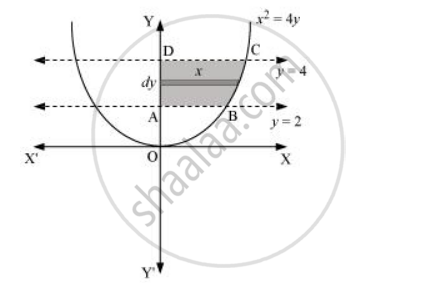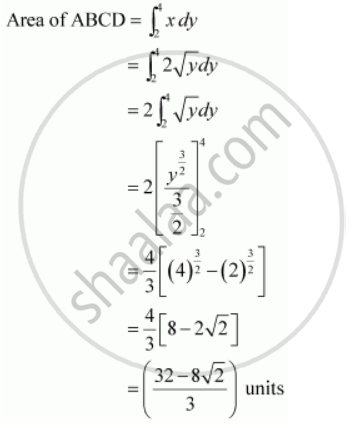Share

# Find the Area of the Region Bounded by X2 = 4y, Y = 2, Y = 4 and the Y-axis in the First Quadrant. - CBSE (Science) Class 12 - Mathematics

#### Question

Find the area of the region bounded by x2 = 4yy = 2, y = 4 and the y-axis in the first quadrant.

#### SolutionThe area of the region bounded by the curve, x2 = 4yy = 2, and y = 4, and the y-axis is the area ABCD.Is there an error in this question or solution?

#### APPEARS IN

NCERT Solution for Mathematics Textbook for Class 12 (2018 to Current)
Chapter 8: Application of Integrals
Q: 3 | Page no. 366
Solution Find the Area of the Region Bounded by X2 = 4y, Y = 2, Y = 4 and the Y-axis in the First Quadrant. Concept: Area Under Simple Curves.
S